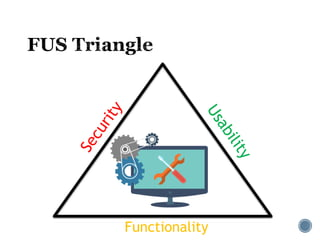Successfully reported this slideshow.

# Basics of Cryptography

0

Share×
1 of 25
1 of 25

# Basics of Cryptography

0

Share

A very understanding of cryptography. Presented at Null Meet Pune (May 2017).

A very understanding of cryptography. Presented at Null Meet Pune (May 2017).

## More Related Content

### Related Books

Free with a 14 day trial from Scribd

See all

### Related Audiobooks

Free with a 14 day trial from Scribd

See all

### Basics of Cryptography

1. 1. For the beginners, by a beginner
2. 2.  Security Enthusiast  Broke lock of my own bag.  Start many things, finish nothing (mostly)  Threat Researcher @ Symantec  B&B  Talking at badboy16a@gmail.com  Broadcasting @_badbot
3. 3. Functionality Systems
4. 4.  Encoding  Encryption  Obfuscation  Cryptography  Cryptanalysis  Steganography
5. 5.  Transposition  scytale  Substitution  Caesar cipher  Enigma  Shannon’s law of secrecy  PGP
6. 6.  Messages is split in to fixed size blocks.
7. 7. Block Encryption
8. 8.  Electronic CodeBook (ECB)  Cipher Block Chaining (CBC)  Cipher FeedBack(CFB)  Output Feedback(OFB)  Counter (CTR)  Galois Counter Mode (GCM)
9. 9.  Required to align data to Block length.  Bit Padding  Byte Padding  Zero Padding  ANSIx.923  ISO-10126  PKCS#7  ISO/IEC 7816-4
10. 10.  One way function.  Arbitrary length input, fixed length output.  Examples:  CRC(16/32)  MD(4/5)  SHA(1/2/3/4)
11. 11.  Integers  Prime  Co-prime  Modulus  Congruenc e  Group  Modulus (M)  Operation  Identity  Inverse  Generator  Ring  Order (M-1) iff M is prime.
12. 12.  Diffie-Hellman Key Exchange  Alice and Bob agree to use a modulus p = 23 and generator g = 5.  Alice chooses a secret integer a = 6, then sends Bob A = ga mod p  A = 56 mod 23 = 8  Bob chooses a secret integer b = 15, then sends Alice B = gb mod p  B = 515 mod 23 = 19  Alice computes s = Ba mod p  s = 196 mod 23 = 2  Bob computes s = Ab mod p  s = 815 mod 23 = 2  Alice and Bob now share a secret (the number 2).
13. 13.  Based on intractability principle  Multiplying two large integers is easy  Finding prime factors of large integers is an intractable problem.  Computations are performed in “Group” modulo M.  M being a very large prime.  2 keys are generated simultaneously.  Inverse of each other (modulo M).  One encrypts (Public Key)  Other decrypts (Private Key)
14. 14. Encryption (P=5) C = Pe mod n 53 % 33 = 125 % 3 = 26 Decryption P = Cd mod n 267 % 33 = 8031810176 % 33 = 5 OR 267 % 33 = 26 (3+3+1) % 33 = (263%33)* (263%33)* (26%33) = 5 Key Generation Select 2 primes: p,q p = 11, q = 3 Calculate: n = p*q n = 33 Calculate: Φ(n) = (p-1)*(q-1) Φ(n) = 20 Choose e : gcd(e, Φ(n)) = 1, (e,n) is public key e = 3; Kpub = (3,33) Find d : e*d = 1 mod Φ(n), (d,n) is private key d = 7, Kpriv = (7,33)

### Editor's Notes

• PGP Later
• https://www.digicert.com/images/iot/IoTSecuritySolutionGraphic_400x400.png
• DES:
by IBM, Lucifer, NSA?
Why not 2DES? Meet-in-the-middle
• Symmetric key must be shared before use.
• Encrypts one bit/byte.
• Linear Feedback Shift Register
Designed to be implemented in H/W (A5/1), CSS
Initial State, Key, Tap Points
• DES Block size: 64 bits, Keysize: 56b (+8b parity)
AES Block Size: 64 bits, Key size: 64, 128, 256
• GCM provides authentication also.
• Must be identifiable after decryption
Ciphertext Stealing

• SHAttered
Fuzzy hashing.
• Primality Test
Multiplicative Group
• Multiplicative group
• RSA??
Extended Euclid,s Algorithm
e is small, usually 65537.
• Trust = Sign their public key.
• The Irony